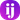Home /

# Sieve of Eratosthenes

What is the Sieve of Eratosthenes? It seems like such a fancy name for something. Wikipedia tells us that Eratosthenes was a Greek mathematician. Wow, cool. Apparently the ancient greeks were good at math :smile:

A prime number sieve is an algorithm to find prime numbers. A prime number, of course, is a number that is only divisible by itself and 1. The first couple primes are: 2, 3, 5, and 7. 4, for example, is not a prime because it is divisible by 1, 2, and 4 which means that it is not a prime number.

The Sieve of Eratosthenes is an efficient way to find small prime numbers. Another common algorithm would be Fermat's Little Theorem (We don't talk about his big theorem :laughing:).

Here are the steps for Eratosthenes algorithm:

1. Create a list n of numbers from 2 to p
2. Set p equal to 2 (the smallest prime number)
3. Go through the list n by multiples of p and mark each number (except for p)
4. Then, find the first greatest number not marked, if there is no number stop, if there is, repeat step 3

Heres a fun gif of how this works (thanks wikipedia):How do we do this with ruby?

I will show you one way. This solution could definitely use some performance optimizations.

First, we want to get the user's input:

`.css-1baulvz{display:inline-block;}puts "Enter the end of the range:"end_of_range_ = gets.chomp.to_i`

With this we can create an array of numbers:

`n = (2..end_of_range).to_a`

Now we need to set up some variables to keep track of what numbers we have checked and what numbers we need to check next.

`# 2 is the smallest prime, we always start with itp = 2numbers_to_remove = []primes_used = []sieve_is_running = true`

Now here is the meat of the program. We want to run a loop that will end when we have searched through the whole array, `n`, and have removed all non-primes. We will use a while loop to get things going.

`while(sieve_is_running)end`

The first thing that we need to do is add each number that we need to remove to an array. This is where I got caught up at first. I started by removing the numbers as I went but this will make skip numbers that we need to check!

`while(sieve_is_running)  n.each do |num|    numbers_to_remove << num * p  end  puts numbers_to_remove.inspect  # If the user entered ten you will get:  # => [4, 6, 8, 10]end`

Next, we need to actually remove the numbers from our original array.

`while(sieve_is_running)  n.each do |num|    numbers_to_remove << num * p  end  numbers_to_remove.each do |num|    n.delete(num)  end  puts n.inspect  # Again, if user enters 10:  # => [2,3,5,7,9]end`

After this, we need to keep track of the prime that we have used and find the next prime to check the array with:

`while(sieve_is_running)  n.each do |num|    numbers_to_remove << num * p  end  numbers_to_remove.each do |num|    n.delete(num)  end  # keep track of which primes we have used  primes_used << p  # find the next number to use as P  n.each do |num|    if !primes_used.include? num      p = num      break    end  endend`

Now this works! The only problem is that our while loop will continue to run forever. We need to tell it to stop by checking if the length of the `primes_used` array and `n` are the same and set `sieve_is_running = false`

`while(sieve_is_running)  n.each do |num|    numbers_to_remove << num * p  end  numbers_to_remove.each do |num|    n.delete(num)  end  # keep track of which primes we have used  primes_used << p  # find the next number to use as P  n.each do |num|    if !primes_used.include? num      p = num      break    elsif primes_used.size == n.size      sieve_is_running = false    end  endend`

And there it is! Heres the whole script for an overview:

`# get user inputputs "Enter the end of the range"end_of_range = gets.chomp.to_in = (2..end_of_range).to_ap = 2numbers_to_remove = []primes_used = []sieve_is_running = truewhile(sieve_is_running)  n.each do |num|    numbers_to_remove << num * p  end  numbers_to_remove.each do |num|    n.delete(num)  end  # keep track of which primes we have used  primes_used << p  # find the next number to use as P  n.each do |num|    if !primes_used.include? num      p = num      break    elsif primes_used.size == n.size      sieve_is_running = false    end  endendputs n.inspect# if user enters 10# => [2,3,5,7]`

Cool! One thing that I have found with this script is that if you enter 1000 as the `end_of_range` it will take a while. Some optimizations that you could make in the future is to not add numbers to `numbers_to_remove` that you know are not in n. An example of this would be:

If the use enter 10 and `p` is 2, the number that will currently get put into `numbers_to_remove` are: [4,6,8,10,12,14,16,18,20]. We know that our array `n` does not contain numbers any larger than 10, so we can go ahead an only insert numbers into `numbers_to_remove` that are less than or equal to `end_of_range`.

Are there any optimizations that you can think of that would make this run faster? Let me know! I would love to hear any feedback :smile: